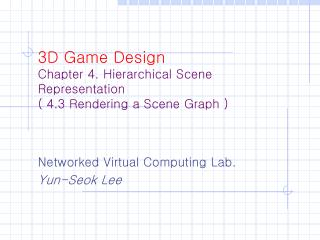# 3D Game Design Chapter 4. Hierarchical Scene Representation ( 4.3 Rendering a Scene Graph ) - PowerPoint PPT PresentationDownload Presentation3D Game Design Chapter 4. Hierarchical Scene Representation ( 4.3 Rendering a Scene Graph )

3D Game Design Chapter 4. Hierarchical Scene Representation ( 4.3 Rendering a Scene Graph )Download Presentation## 3D Game Design Chapter 4. Hierarchical Scene Representation ( 4.3 Rendering a Scene Graph )

- - - - - - - - - - - - - - - - - - - - - - - - - - - E N D - - - - - - - - - - - - - - - - - - - - - - - - - - -
##### Presentation Transcript

1. 3D Game DesignChapter 4. Hierarchical Scene Representation( 4.3 Rendering a Scene Graph ) Networked Virtual Computing Lab. Yun-Seok Lee

2. Basic Concept • View frustum • The portion of the world to be viewed • The world bounding volume is used to determine whether the object is culled or not. • Geometry classes could provide a Boolean flag indicating whether the world data is current or not.

3. 1. Culling by Spheres • View frustum plane is specified by • N•X = d • The culling condition • N•C– d < -r • N : unit-length vector that points to the interior of the frustum • C : center of the bounding sphere • r : radius of the sphere • The left side of the inequality is the signed distance from C to plane.

4. 2. Culling by Oriented Boxes • A box is culled. • If all its vertices are outside the plane. • Vertices, distance and • The culling condition • The test is identical to that of sphere, except that r is known for the sphere but must be calculated for each test of a box. radius

5. 3. Culling by Capsules • A capsule • A radius r > 0 • A parameterized line segment. P + tD, where D≠0 and t∈[0,1]. • The signed distances from plane to end points. • The culling condition • if • if // culled • else if • if // culled

6. 4. Culling by Lozenges • A lozenge • A radius r > 0 • A parameterized rectangle P + sE0 + tE1, where E0 ≠ 0, E1≠0, E0•E1=0, (s,t)∈[0,1]2. • The four rectangle corners • P00 = P, P10 = P+ E0, P01 = P+ E1, P11 = P+ E0 + E1. • The signed distances • If any of the signed distances are nonnegative, then the lozenge is not culled. • The distance of the nearest corner to the plane is nonnegative, then the lozenge is not culled.

7. 5. Culling by Cylinders • A cylinder • A radius r > 0. a height h∈[0,∞]. • A parameterized segment C + tW, where |W|=1 and t∈[-h/2, h/2]. • U, VandW is an orthonormal set. • , and • The culling condition • if • if // culled • else if • If or • If / culled

8. 6. Culling by Ellipsoids • An ellipsoids • , where, M is a positive definite matrix. • If C + sN is outside the frustum plane, an ellipsoid is outside. • A radius of an ellipsoid • The points X that project to the end points of the interval must occur where the normals to the ellipsoid are parallel to N. • -> -> -> • -> • // culled